# Data Augmentations

## Pixellation

`output = upsample(boxFilter(input))`

The data augmentation blurs the image with a box filter first where each output value is the average of the pixel values in the filter window resulting in a downsampled version of the input image. A resizing operation is needed to restore the resolution using a nearest-neighbour upsampling. This results in the same colour value copied over in the original image in the corresponding blur window. In summary, the box filter is slided across the image with each pixel value changed to the average in the filter window.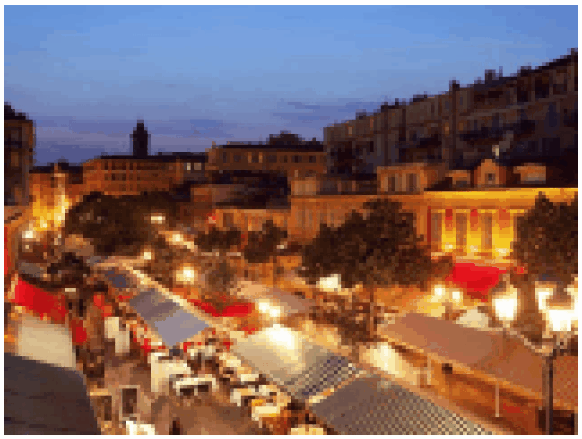```#sample code:
#register augmentation
rep.AnnotatorRegistry.register_augmentation(name="AugPixellateExp", augmentation=rep.Augmentation.from_node("omni.replicator.core.AugPixellateExp", kernelSize=8))
#augment annotator product
ldr_color = ldr_color.augment("AugPixellateExp")
```

## Motion Blur

`output = conv2D(input, blurfilter)`

Motion blur is simulated using a 2D kernel (also called filter) but the user can choose the angle and direction — whether it is going forward or backward — as well as the kernel size. The kernel is then used to convolve the 2D image to produce motion blur like effects.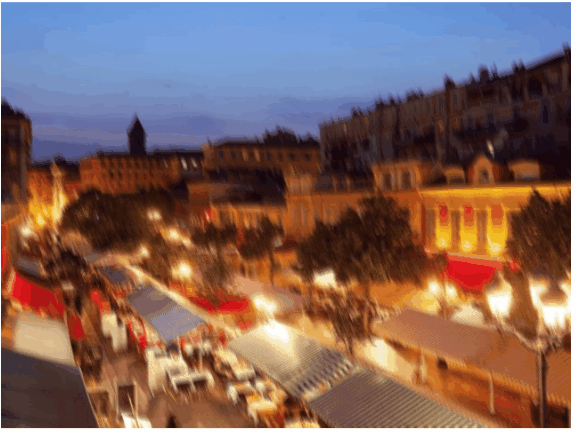```#sample code:
#register augmentation
rep.AnnotatorRegistry.register_augmentation(name="AugMotionBlurExp", augmentation=rep.Augmentation.from_node("omni.replicator.core.AugMotionBlurExp", alpha=0.7, kernelSize=11))
#augment annotator product
ldr_color = ldr_color.augment("AugMotionBlurExp")
```

## Glass Blur

`output = glassBlur(input)`

To simulate glass blur, we choose a delta parmeter, which captures the maximum window size inside which two pixel locations are chosen and their colour values swapped.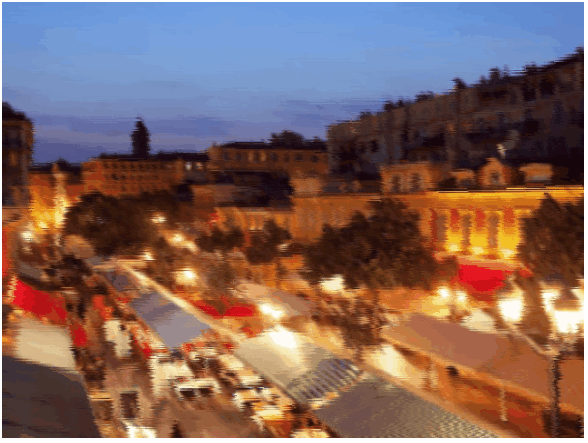```#sample code:
#register augmentation
rep.AnnotatorRegistry.register_augmentation(name="AugGlassBlur", augmentation=rep.Augmentation.from_function(rep.aug_glass_blur, data_out_shape=(-1, 4), delta=rep.random.choice([1, 2, 3, 4])))
#augment annotator product
ldr_color = ldr_color.augment("AugGlassBlur")
```

## Rand Conv

`output = conv2D(input, randomFilter)`

The image is convolved with a random k x k filter generated on the fly. The weights are sampled from classic He-initialisation used in deep learning. It provides various colour changes the image can undergo.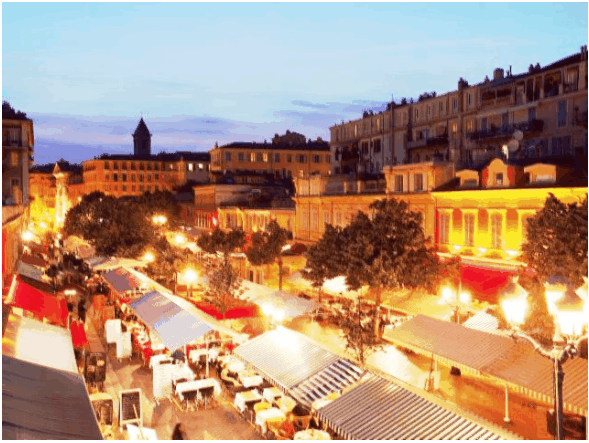```#sample code:
#register augmentation
rep.AnnotatorRegistry.register_augmentation(name="AugConv2dExp", augmentation=rep.Augmentation.from_node("omni.replicator.core.AugConv2dExp", alpha=0.7, kernelWidth=3))
#augment annotator product
ldr_color = ldr_color.augment("AugConv2dExp")
```

## RGB ↔ HSV

Converts the standard RGB colour palette into a polar HSV colour palette. Various augmentations can then be applied in this space and converted back to RGB.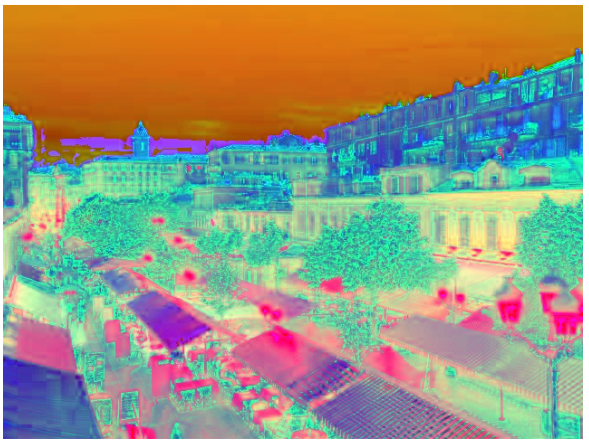```#sample code:
#register augmentation
rep.AnnotatorRegistry.register_augmentation(name="AugRGBtoHSV", augmentation=rep.Augmentation.from_function(rep.aug_rgb_to_hsv, data_out_shape=(-1, 4)))

rep.AnnotatorRegistry.register_augmentation(name="AugHSVtoRGB", augmentation=rep.Augmentation.from_function(rep.aug_hsv_to_rgb, data_out_shape=(-1, 4)))

#augment annotator product
ldr_color = ldr_color.augment("AugRGBtoHSV")
ldr_color = ldr_color.augment("AugHSVtoRGB")
```

## CutMix

`output = ( 1 - mask) inputImage + mask randomImage`

The augmentation takes in a random rectangular patch from another image and superimposes it on the input image. The rectangular patch is encoded in a binary mask where the pixels belonging to the rectangle have a mask value of 1 and 0 otherwise.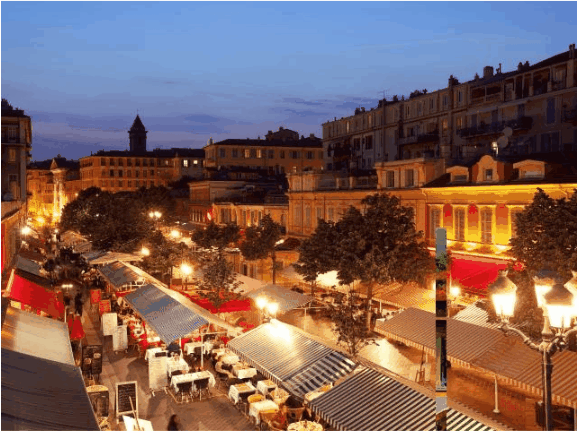```#sample code:
#register augmentation
rep.AnnotatorRegistry.register_augmentation(name="AugCutMixExp", augmentation=rep.Augmentation.from_node("omni.replicator.core.AugCutMixExp", folderpath="/folder/to/random/ims/"))
#augment annotator product
ldr_color = ldr_color.augment("AugCutMixExp")
```

## Random Blend

`output = ( 1 - randomFactor) inputImage + randomFactor randomImage`

Two images are blended in with a random blending factor to generate an image that contains the data from both images. This is basically linearly interpolating two images.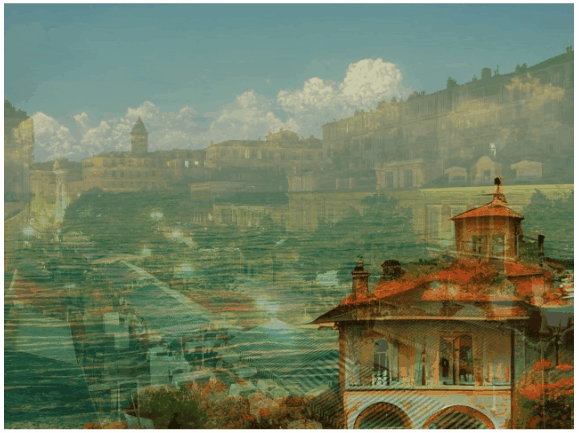```#sample code:
#register augmentation
rep.AnnotatorRegistry.register_augmentation(name="AugImgBlendExp", augmentation=rep.Augmentation.from_node("omni.replicator.core.AugImgBlendExp", folderpath="/folder/to/random/ims/"))
#augment annotator product
ldr_color = ldr_color.augment("AugImgBlendExp")
```

## Background Randomization

`output = ( 1 - backgroundAlpha) inputImage + backgroundAlpha randomImage`

The blank background of an image is replaced with an arbitrary image. The alpha channel is used to combine the images, with precise alpha compositing at the edges between foreground and background. Provided a folder of background images of arbitrary size, each execution of the node samples a preloaded image from the folder.```#sample code:
#register augmentation
rep.AnnotatorRegistry.register_augmentation(name="AugBgRandExp", augmentation=rep.Augmentation.from_node("omni.replicator.core.AugBgRandExp", folderpath="/folder/to/background/ims/"))
#augment annotator product
ldr_color = ldr_color.augment("AugBgRandExp")
```

## Contrast

`output = inputImage*(1-enhancement)+mean(inputImage)*enhancement`

Standard color augmentation to alter image contrast.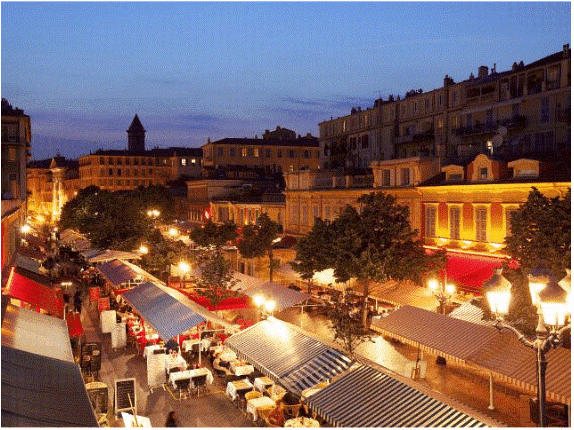```#sample code:
#register augmentation
rep.AnnotatorRegistry.register_augmentation(name="AugContrastExp", augmentation=rep.Augmentation.from_node("omni.replicator.core.AugContrastExp", contrastFactorIntervalMin=0.2,contrastFactorIntervalMax=5))
#augment annotator product
ldr_color = ldr_color.augment("AugContrastExp")
```

## Brightness

`output = inputImage +brightnessFactor`

Standard color augmentation to alter image brightness.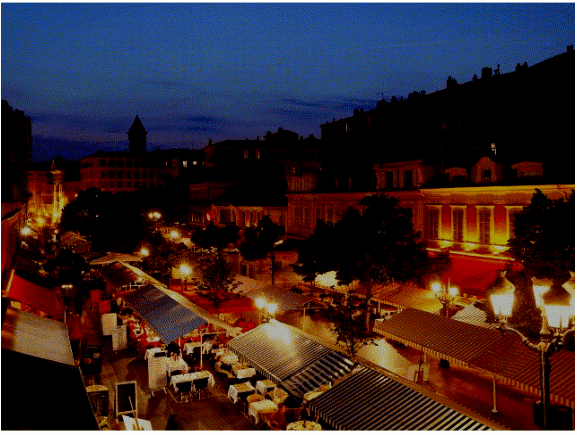```#sample code:
#register augmentation
rep.AnnotatorRegistry.register_augmentation(name="AugBrightness", augmentation=rep.Augmentation.from_function(rep.aug_brightness, data_out_shape=(-1, 4), seed=31, brightness_factor=rep.distribution.uniform(-100, 100)))
#augment annotator product
ldr_color = ldr_color.augment("AugBrightness")
```

## Rotate

`output = rotate(inputImage, angle)`

Random rotation of the image about its center.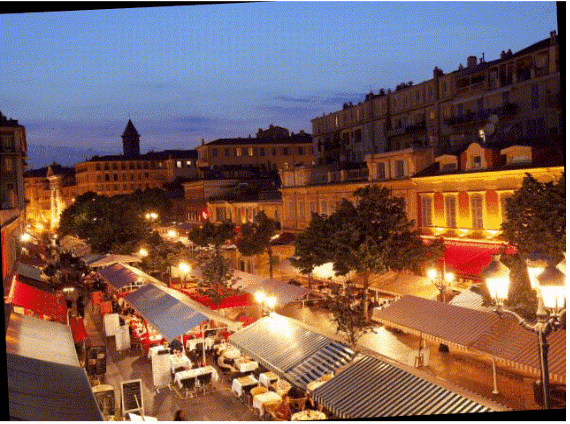```#sample code:
#register augmentation
rep.AnnotatorRegistry.register_augmentation(name="AugRotateExp",augmentation=rep.Augmentation.from_node("omni.replicator.core.AugRotateExp", rotateDegrees=40.0))
#augment annotator product
ldr_color = ldr_color.augment("AugRotateExp")
```

## Crop and Resize

`output = resize(crop(inputImage, xmin, xmax, ymin, xmax))`

Random crop of the image in each x and y direction, and resize (i.e. zoom) back to original shape using warp.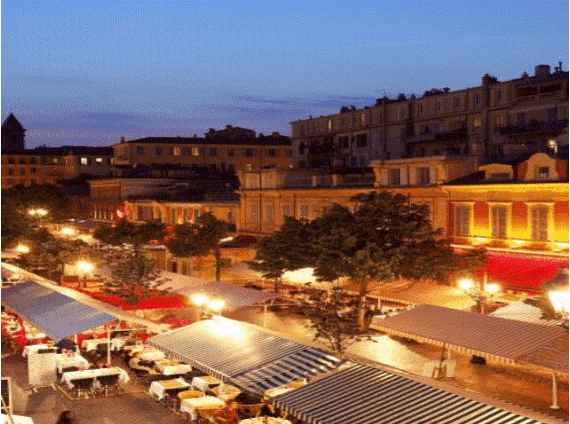```#sample code:
#register augmentation
rep.AnnotatorRegistry.register_augmentation(name="AugCropResizeExp", augmentation=rep.Augmentation.from_node("omni.replicator.core.AugCropResizeExp", minPercent=0.5))
#augment annotator product
ldr_color = ldr_color.augment("AugCropResizeExp")
```

`output = exp(1+gain*(cutoff-inputImage))^-1`

Provided a cutoff and a gain, adjust the sigmoid of the image.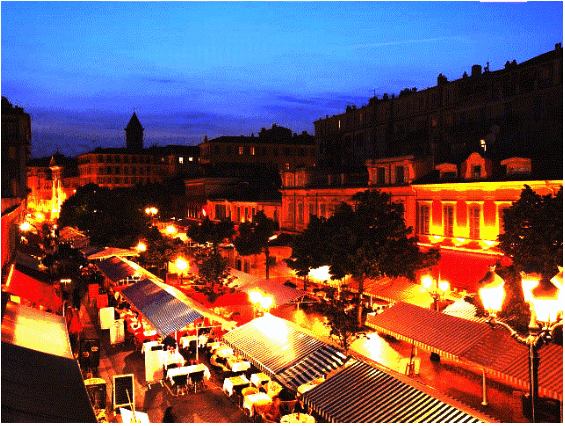```#sample code:
#register augmentation
#augment annotator product
```

## Speckle Noise

`output = inputImage + (sigma * noiseArray ⊙ inputImage)`

Provided a noise scaling factor sigma, add speckle noise to each pixel of the image.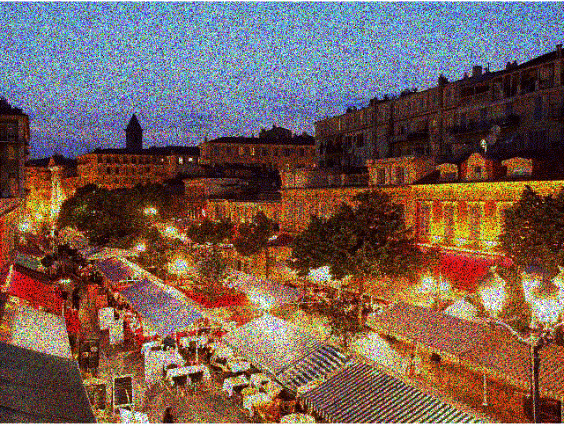```#sample code:
#register augmentation
rep.AnnotatorRegistry.register_augmentation(name="AugSpeckleNoise", augmentation=rep.Augmentation.from_function(rep.aug_speckle_noise, data_out_shape=(-1, 4),sigma=rep.distribution.uniform(0, 5)))
#augment annotator product
ldr_color = ldr_color.augment("AugSpeckleNoise")
```

## Shot Noise

`output = inputImage + noiseArray ⊙ √(inputImage)/√(sigma)`

Provided a noise scaling factor sigma, add shot noise to each pixel of the image.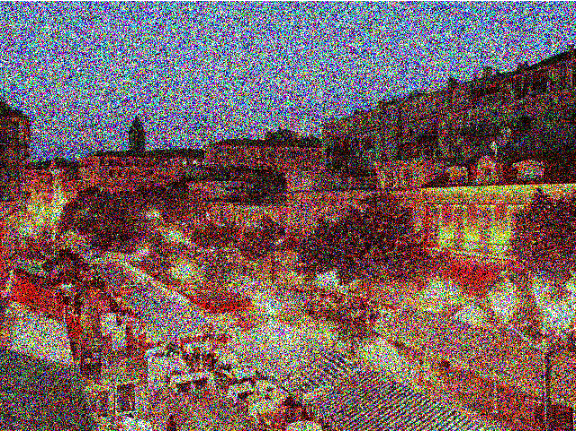```#sample code:
#register augmentation
rep.AnnotatorRegistry.register_augmentation(name="AugShotNoise",augmentation=rep.Augmentation.from_function(rep.aug_shot_noise, data_out_shape=(-1, 4), sigma=rep.distribution.normal(20, 5)))
#augment annotator product
ldr_color = ldr_color.augment("AugShotNoise")
```

Notes

• All augmentations with suffix -Exp do not support replicator distribution sampling (e.g. `rep.distribution.normal(20, 5)`. For these nodes, either specify the distribution parameters if provided or sample externally.